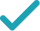Type a math problemFactorSolution Steps
Steps Using Direct Factoring Method
Solution Steps
Factor out .
Consider . Factor the expression by grouping. First, the expression needs to be rewritten as . To find and , set up a system to be solved.
Since is positive, and have the same sign. Since is positive, and are both positive. List all such integer pairs that give product .
Calculate the sum for each pair.
The solution is the pair that gives sum .
Rewrite as .
Factor out in the first and in the second group.
Factor out common term by using distributive property.
Rewrite the complete factored expression.
EvaluateGraphGiving is as easy as 1, 2, 3
Get 1,000 points to donate to a school of your choice when you join Give With Bing
2\left(x^{2}+8x+12\right)
Factor out 2.
a+b=8 ab=1\times 12=12
Consider x^{2}+8x+12. Factor the expression by grouping. First, the expression needs to be rewritten as x^{2}+ax+bx+12. To find a and b, set up a system to be solved.
1,12 2,6 3,4
Since ab is positive, a and b have the same sign. Since a+b is positive, a and b are both positive. List all such integer pairs that give product 12.
1+12=13 2+6=8 3+4=7
Calculate the sum for each pair.
a=2 b=6
The solution is the pair that gives sum 8.
\left(x^{2}+2x\right)+\left(6x+12\right)
Rewrite x^{2}+8x+12 as \left(x^{2}+2x\right)+\left(6x+12\right).
x\left(x+2\right)+6\left(x+2\right)
Factor out x in the first and 6 in the second group.
\left(x+2\right)\left(x+6\right)
Factor out common term x+2 by using distributive property.
2\left(x+2\right)\left(x+6\right)
Rewrite the complete factored expression.
2x^{2}+16x+24=0
Quadratic polynomial can be factored using the transformation ax^{2}+bx+c=a\left(x-x_{1}\right)\left(x-x_{2}\right), where x_{1} and x_{2} are the solutions of the quadratic equation ax^{2}+bx+c=0.
x=\frac{-16±\sqrt{16^{2}-4\times 2\times 24}}{2\times 2}
All equations of the form ax^{2}+bx+c=0 can be solved using the quadratic formula: \frac{-b±\sqrt{b^{2}-4ac}}{2a}. The quadratic formula gives two solutions, one when ± is addition and one when it is subtraction.
x=\frac{-16±\sqrt{256-4\times 2\times 24}}{2\times 2}
Square 16.
x=\frac{-16±\sqrt{256-8\times 24}}{2\times 2}
Multiply -4 times 2.
x=\frac{-16±\sqrt{256-192}}{2\times 2}
Multiply -8 times 24.
x=\frac{-16±\sqrt{64}}{2\times 2}
x=\frac{-16±8}{2\times 2}
Take the square root of 64.
x=\frac{-16±8}{4}
Multiply 2 times 2.
x=\frac{-8}{4}
Now solve the equation x=\frac{-16±8}{4} when ± is plus. Add -16 to 8.
x=-2
Divide -8 by 4.
x=\frac{-24}{4}
Now solve the equation x=\frac{-16±8}{4} when ± is minus. Subtract 8 from -16.
x=-6
Divide -24 by 4.
2x^{2}+16x+24=2\left(x-\left(-2\right)\right)\left(x-\left(-6\right)\right)
Factor the original expression using ax^{2}+bx+c=a\left(x-x_{1}\right)\left(x-x_{2}\right). Substitute -2 for x_{1} and -6 for x_{2}.
2x^{2}+16x+24=2\left(x+2\right)\left(x+6\right)
Simplify all the expressions of the form p-\left(-q\right) to p+q.
x ^ 2 +8x +12 = 0
Quadratic equations such as this one can be solved by a new direct factoring method that does not require guess work. To use the direct factoring method, the equation must be in the form x^2+Bx+C=0.This is achieved by dividing both sides of the equation by 2
r + s = -8 rs = 12
Let r and s be the factors for the quadratic equation such that x^2+Bx+C=(x−r)(x−s) where sum of factors (r+s)=−B and the product of factors rs = C
r = -4 - u s = -4 + u
Two numbers r and s sum up to -8 exactly when the average of the two numbers is \frac{1}{2}*-8 = -4. You can also see that the midpoint of r and s corresponds to the axis of symmetry of the parabola represented by the quadratic equation y=x^2+Bx+C. The values of r and s are equidistant from the center by an unknown quantity u. Express r and s with respect to variable u. <div style='padding: 8px'><img src='https://opalmath.azureedge.net/customsolver/quadraticgraph.png' style='width: 100%;max-width: 700px' /></div>
(-4 - u) (-4 + u) = 12
To solve for unknown quantity u, substitute these in the product equation rs = 12
16 - u^2 = 12
Simplify by expanding (a -b) (a + b) = a^2 – b^2
-u^2 = 12-16 = -4
Simplify the expression by subtracting 16 on both sides
u^2 = 4 u = \pm\sqrt{4} = \pm 2
Simplify the expression by multiplying -1 on both sides and take the square root to obtain the value of unknown variable u
r =-4 - 2 = -6 s = -4 + 2 = -2
The factors r and s are the solutions to the quadratic equation. Substitute the value of u to compute the r and s.